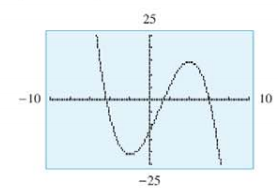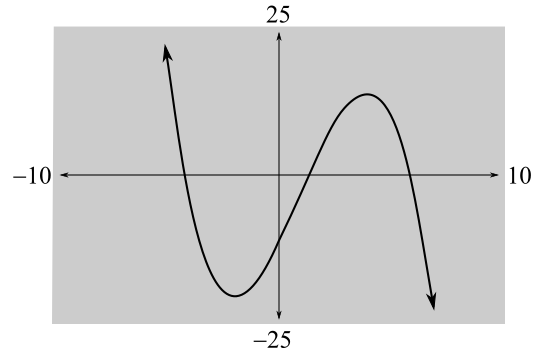Chapter 10.2, Problem 23E### Mathematical Applications for the ...

11th Edition
Ronald J. Harshbarger + 1 other
ISBN: 9781305108042

#### Solutions

Chapter
Section### Mathematical Applications for the ...

11th Edition
Ronald J. Harshbarger + 1 other
ISBN: 9781305108042
Textbook Problem

# In Problems 25 and 26, a function and its graph are given, (a) From the graph, estimate where f   " ( x ) > 0, where f   " ( x ) < 0, and where f   " ( x ) = 0.(h) Use part (a) to decide where f   ' ( x ) has its relative maxima and relative minima.(c) Verify your results in parts (a) and (b) by finding f   ' ( x ) and f"(x) and then graphing each with a graphing utility. f ( x ) = − 1 3 x 3 + x 2 + 8 x − 12(a)

To determine

The region where f(x)>0, f(x)<0 and f(x)=0 in the graph of the function f(x)=13x3+x2+8x12.Explanation

Given Information:

The provided graph of the function f(x)=13x3+x2+8x12 is

Explanation:

Consider the provided graph of the function f(x)=13x3+x2+8x12,

Recall that if f(x)>0 and f(x) is concave up and if f(x)<0 and f(x) is concave down.

From the graph, if x<1 then graph is concave up and if x>1 then the graph is concave down and at point x=1 the graph changes its concavity

(b)

To determine

Where f(x) has relative maxima and relative minima for the provided graph of a function f(x)=13x3+x2+8x12.(c)

To determine

The values of f(x) and f(x), the region where f(x)>0, f(x)<0 and f(x)=0 and where f'(x) has relative maxima and relative minima for the function f(x)=13x3+x2+8x12 and graph each function f(x) and f(x).

### Still sussing out bartleby?

Check out a sample textbook solution.

See a sample solution

#### The Solution to Your Study Problems

Bartleby provides explanations to thousands of textbook problems written by our experts, many with advanced degrees!

Get Started

#### In Exercises 69-74, rationalize the numerator. 69. 2x3

Applied Calculus for the Managerial, Life, and Social Sciences: A Brief Approach

#### Divide: 45x672x3y2

Elementary Technical Mathematics

#### Explain why partial counterbalancing is sometimes necessary.

Research Methods for the Behavioral Sciences (MindTap Course List)